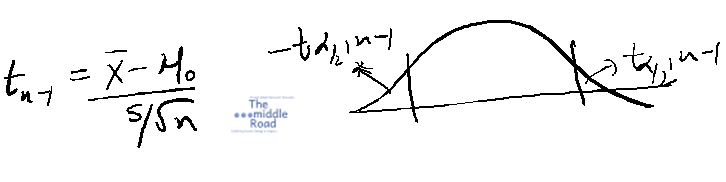Nishant Malhotra Founder of Middle Road OPC Pvt Ltd & The middle Road platform talks about the startup

### Ahoy Ahoy-Ahoy!

Welcome to the Kickass platform enabling social change & impact

# Statistics 5C: Sample Questions

You are here:

### These set of questions are based on hypothesis testing especially for T distribution.

This is a follow up on the modules covered under Statistics significantly t distribution. Check out modules 4 and to the t-distribution module here.Q1. The standard normal distribution has a variance of _______________.

Q2. T- distribution has a mean of zero and a standard deviation _________

• Greater than zero
• Smaller than zero
• Equal to zero

###### Image : Carlos Muza | Unsplash

Q3. Which of the following t-distributions would be closer to a standard normal? DF = Degrees of Freedom

• T-distribution with 20 DF
• T-distribution with 25 DF
• T-distribution with 30 DF

Q4. An agency is hired to find the height of Labrador puppies born in Canada in 2020. You conduct a test on a sample size of 35 puppies. You don’t know the standard deviation of the population mean but know the height of puppies is normally distributed. Which test statistic would you use and why?

Q5. Which distribution has a mean of zero and a standard deviation of 1.

• T-distribution
• Standard Normal Distribution
• Normal Distribution

Q6. As a financial analyst, you are required to select a stock for portfolio optimization. You have a time series data of  stocks for the last 7 years (annualised return) and need a stock with annualised return of 8%. The stock data is from 1970s but the analyst does not get access to this data; has to work with the sample of last seven years. Out of this data of the last 7 years, you select one stock that has an annualised return of 9% with a standard deviation of 4.8%. Is this selection prudent? Use 95% confidence interval to discuss your hypothesis.

This is a two tailed test. The sample mean can be either equal or unequal (less or greater) than the hypothesized population mean. Although the sample size is small, the returns of the stock are normally distributed. Note: returns of stock are assumed to be normally distributed. For a T distribution with N less than 30, the returns must be normal or approximately normally distributed.  The variance of the population mean is unknown, so t distribution test fits the analysis using 7-1 = 6 degrees of freedom. α = 0.05, µ = 8%, n = 7, sample mean is 9%

### H0 µo = 8 | Ha µo = 8

The null hypothesis is always the status quo. Herein we are assuming that the stock has a mean of 8% annualised return. As usual use the p value if p <= 0.05, we reject the null at 5% alpha. You can also use the test statistic test using the confidence interval to check if the hypothesized mean of stock returns lies between the two test statistic points mentioned below. However, for simplification, use the p-value test.  The null hypothesis can be rejected if the t value is greater than 2.447. Use the function T.INV.2T function (p=0.05, DF=6).= 9-8/(4.8/sqrt(7)) =  1/1.814   =  0.551  here sqrt = square root

Use T.DIST.2T function with X the numeric value and 6 as the degree of freedom to get a p value of 0.6. Since this value is higher than 0.05, you cannot reject the null. 2T is for two tailed tests. Note 0.551 is less than 2.447. The null hypothesis cannot be rejected, the analyst is correct in selecting the stock at 95% C.I.

1. 1
2. A Greater than zero
3. C
4. Student t-distribution; the variance of the population mean is unknown. You can also use Z-test for sample size greater than 30 as the sample mean is approximated to the population mean. As the sample size increases the t distribution becomes closer to a normal distribution.
5. Standard Normal Distribution